# Diverging bar chart in ggplot2

## Sample data

The following data frame contains two variables. The first is a categorical variable which represents unique groups (labels, years, …) while the second represents the corresponding value for each label. This data will be used along this tutorial.

``````# Data
set.seed(6)
df <- data.frame(group = LETTERS[1:20],
value = rnorm(20))``````

## Diverging bar graph with `geom_bar`

Diverging bar charts are a type of bar charts which can be used to visualize the spread between values, generally positive and negative.

Default diverging bar chart in ggplot2

In order to create a diverging bar plot in ggplot2 you can use the `geom_bar` function with your data.

``````# install.packages("ggplot2")
library(ggplot2)

ggplot(df, aes(x = group, y = value)) +
geom_bar(stat = "identity",
show.legend = FALSE) + # Remove the legend
xlab("Group") +
ylab("Value")``````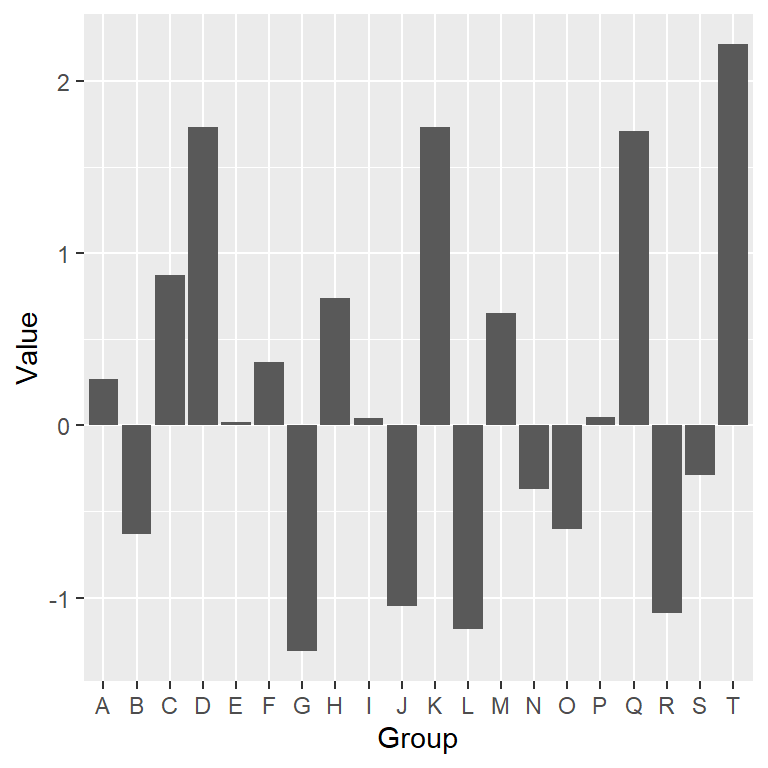Reorder the values

Note that for better visualization it is recommended to reorder the bars based on the value they represent. For that purpose you can use the `reorder` function as shown below.

``````# install.packages("ggplot2")
library(ggplot2)

ggplot(df, aes(x = reorder(group, value), y = value)) +
geom_bar(stat = "identity",
show.legend = FALSE) +
xlab("Group") +
ylab("Value")``````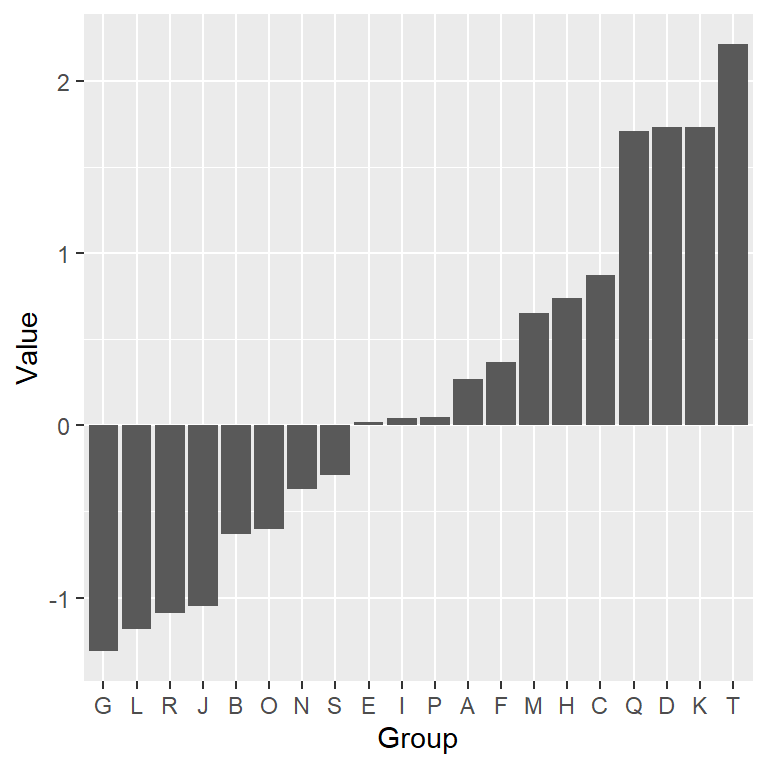Vertical diverging bar chart

Diverging bar charts can both be vertical or horizontal. If you prefer a vertical diverging bar plot change the order of the variables inside `aes` or use `coord_flip` to rotate the axes.

``````# install.packages("ggplot2")
library(ggplot2)

ggplot(df, aes(x = reorder(group, value), y = value)) +
geom_bar(stat = "identity",
show.legend = FALSE) +
xlab("Group") +
ylab("Value") +
coord_flip()``````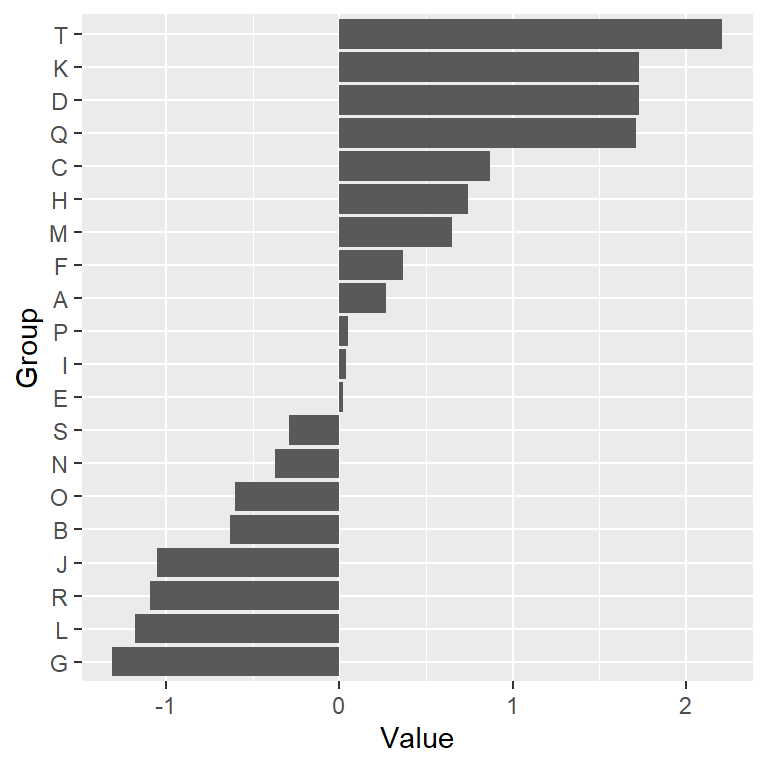## Adding or customizing text labels

You will probably need to rotate the labels of your plot if they are too long or just add additional information to the bars. In this scenario you will need to use the arguments of the `theme` function, modify the axes limits or use the `geom_text` function for adding additional texts, as shown in the following examples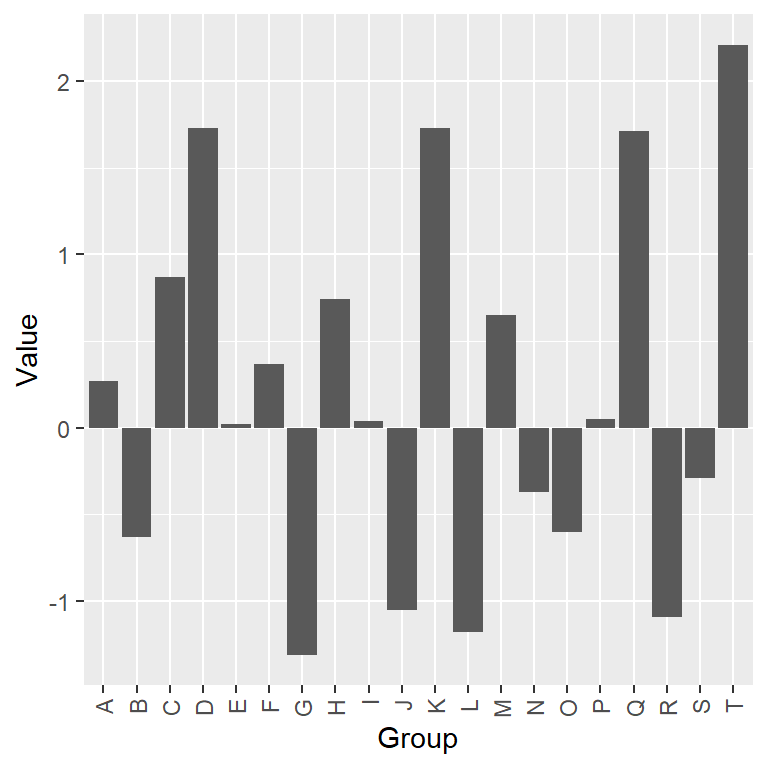Flipping the names of the categorical variable

``````# install.packages("ggplot2")
library(ggplot2)

ggplot(df, aes(x = group, y = value)) +
geom_bar(stat = "identity",
show.legend = FALSE) +
xlab("Group") +
ylab("Value") +
theme(axis.text.x = element_text(angle = 90,
hjust = 1,
vjust = 0.5))``````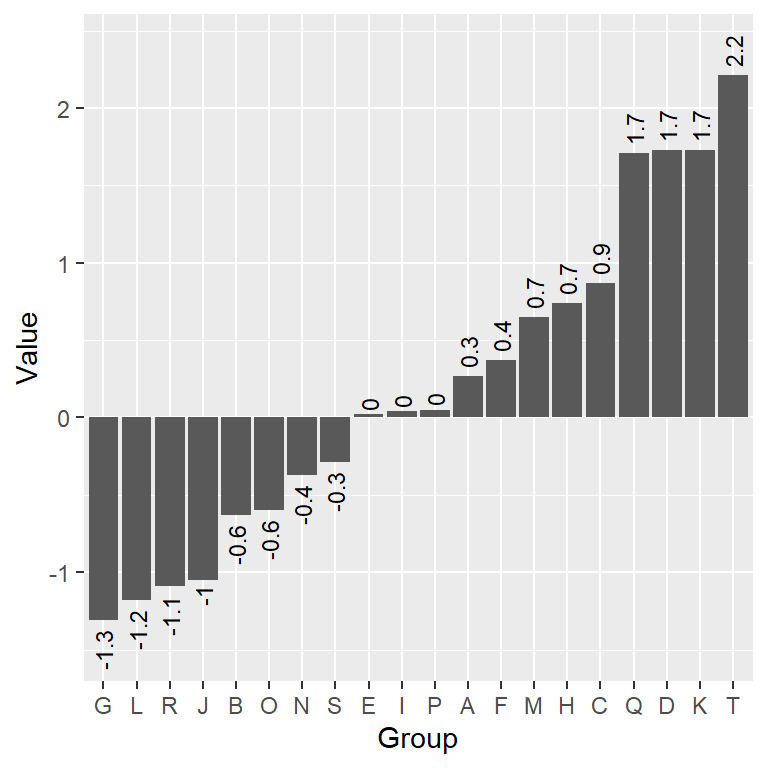Adding text for each bar (vertical text)

``````# install.packages("ggplot2")
library(ggplot2)

ggplot(df, aes(x = reorder(group, value), y = value)) +
geom_bar(stat = "identity",
show.legend = FALSE) +
geom_text(aes(label = round(value, 1),
angle = 90,
hjust = ifelse(value < 0, 1.25, -0.25),
vjust = 0.5),
size = 3) +
xlab("Group") +
ylab("Value") +
scale_y_continuous(limits = c(min(df\$value) - 0.2,
max(df\$value) + 0.2))``````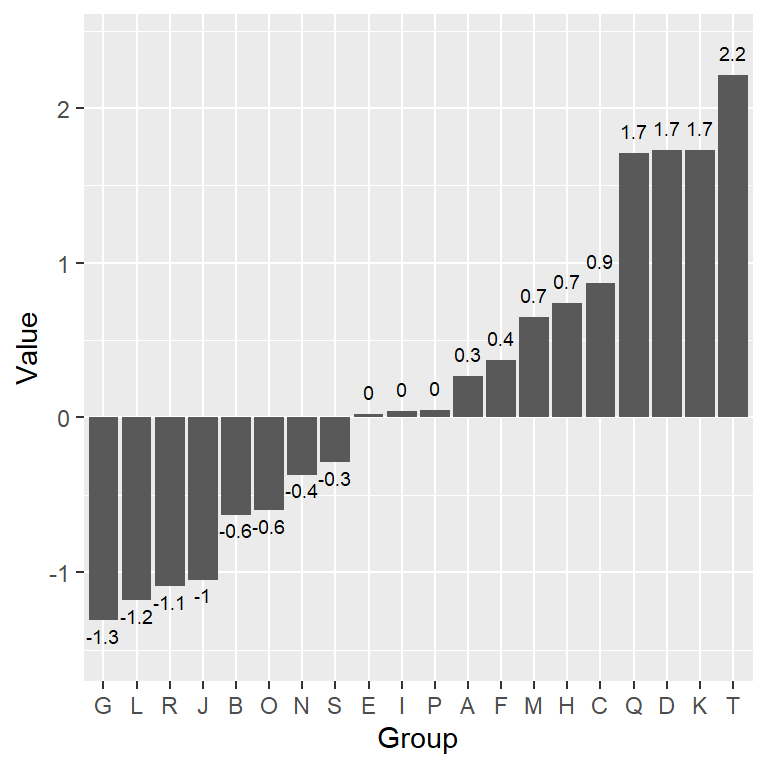Adding text for each bar (horizontal text)

``````# install.packages("ggplot2")
library(ggplot2)

ggplot(df, aes(x = reorder(group, value), y = value)) +
geom_bar(stat = "identity",
show.legend = FALSE) +
geom_text(aes(label = round(value, 1),
hjust = 0.5,
vjust = ifelse(value < 0, 1.5, -1)),
size = 2.5) +
xlab("Group") +
ylab("Value") +
scale_y_continuous(limits = c(min(df\$value) - 0.2,
max(df\$value) + 0.2))``````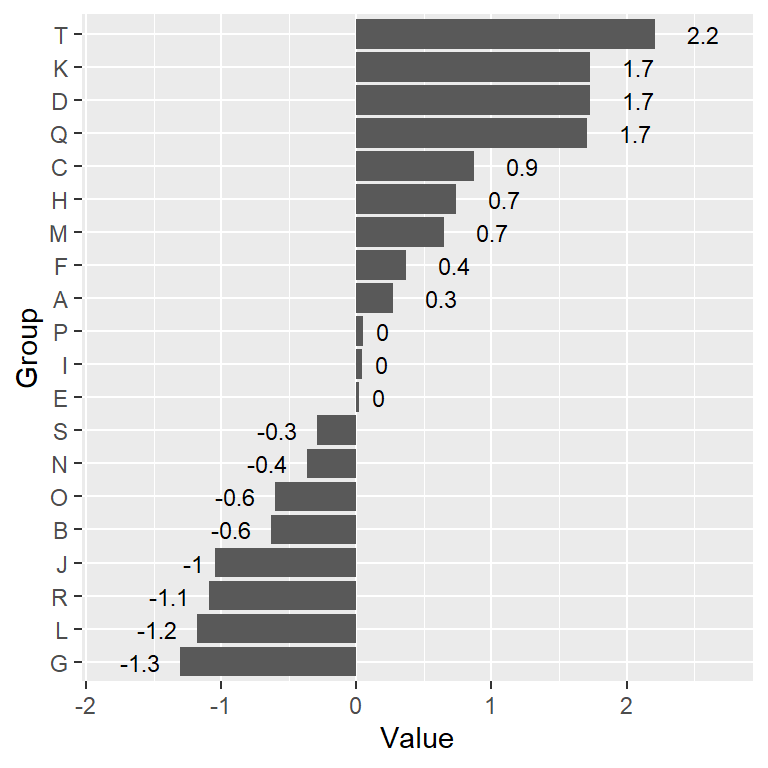Adding text for each bar (vertical plot)

``````# install.packages("ggplot2")
library(ggplot2)

ggplot(df, aes(x = reorder(group, value), y = value)) +
geom_bar(stat = "identity",
show.legend = FALSE) +
geom_text(aes(label = round(value, 1),
hjust = ifelse(value < 0, 1.5, -1),
vjust = 0.5),
size = 3) +
xlab("Group") +
ylab("Value") +
coord_flip() +
scale_y_continuous(breaks= seq(-2, 2, by = 1),
limits = c(min(df\$value) - 0.5,
max(df\$value) + 0.5))``````

## Color customization

By default, the bars of the diverging bar chart are dark gray. However, it is very easy to customize the fill color of the bars and its border color in several ways.

Overriding the default bars color

In order to change the background and the border color for all the bars just pass the desired colors to `fill` and `color` arguments of `geom_bar`, respectively.

``````# install.packages("ggplot2")
library(ggplot2)

ggplot(df, aes(x = reorder(group, value), y = value)) +
geom_bar(stat = "identity",
show.legend = FALSE,
fill = 4,          # Background color
color = "white") + # Border color
xlab("Group") +
ylab("Value")``````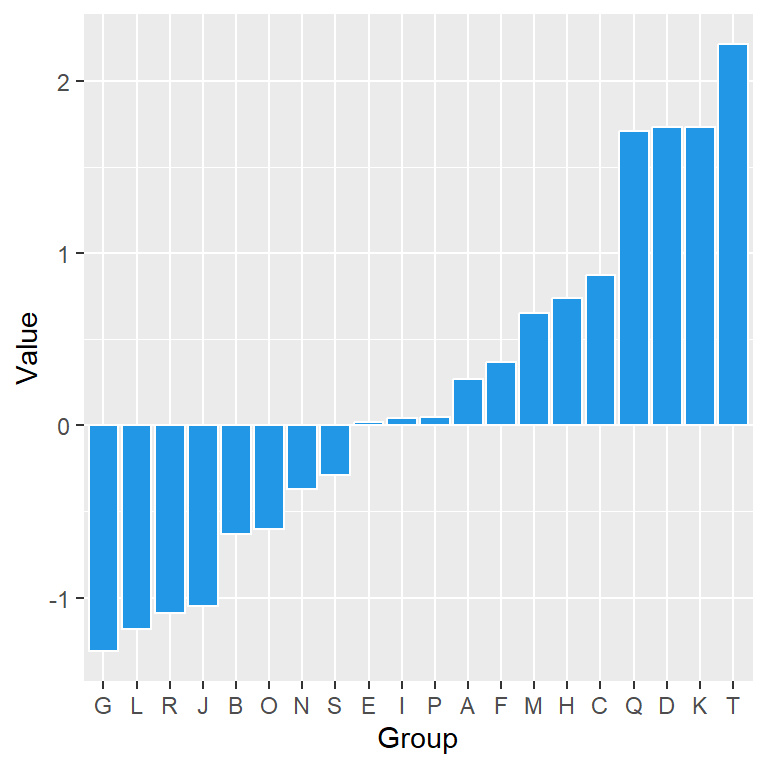Color based on value

It is very common to set a different color based on the break point. To achieve a different color you can create a new categorical variable based on the desired value and pass it to the `fill` argument.

``````# install.packages("ggplot2")
library(ggplot2)

# Color based on value
color <- ifelse(df\$value < 0, "pink", "lightblue")

ggplot(df, aes(x = reorder(group, value), y = value)) +
geom_bar(stat = "identity",
show.legend = FALSE,
fill = color,      # Background color
color = "white") + # Border color
xlab("Group") +
ylab("Value")``````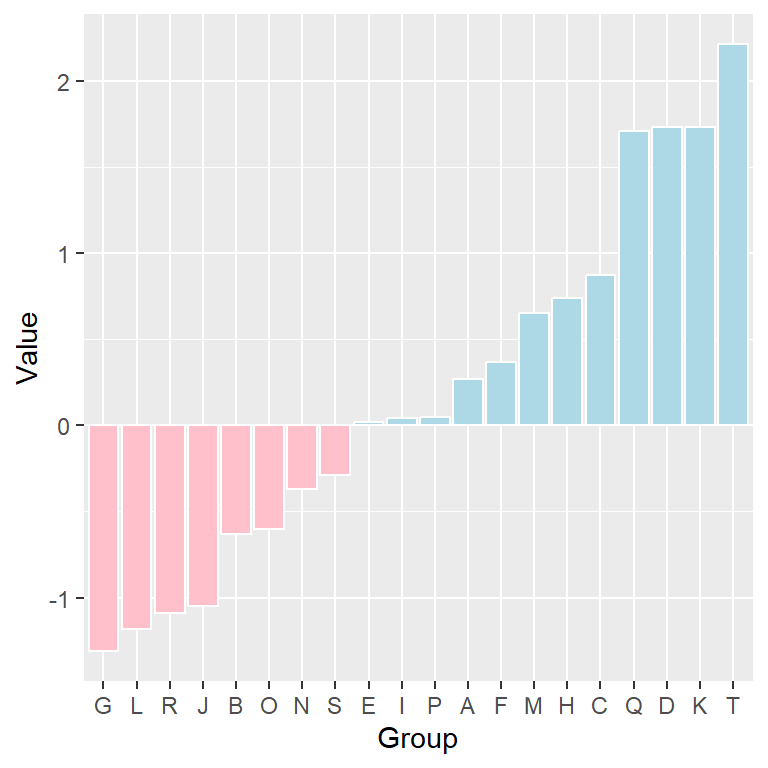Note that you can also pass a numerical variable to `fill`. The default gradient colors of the bars can be modified making use of the `scale_fill_gradient` functions.

``````# install.packages("ggplot2")
library(ggplot2)

ggplot(df, aes(x = reorder(group, value), y = value)) +
geom_bar(stat = "identity",
show.legend = FALSE,
aes(fill = value),  # Background color
color = "gray30") + # Border color
xlab("Group") +
ylab("Value") +
mid = "aliceblue",
high = "#6495ED")``````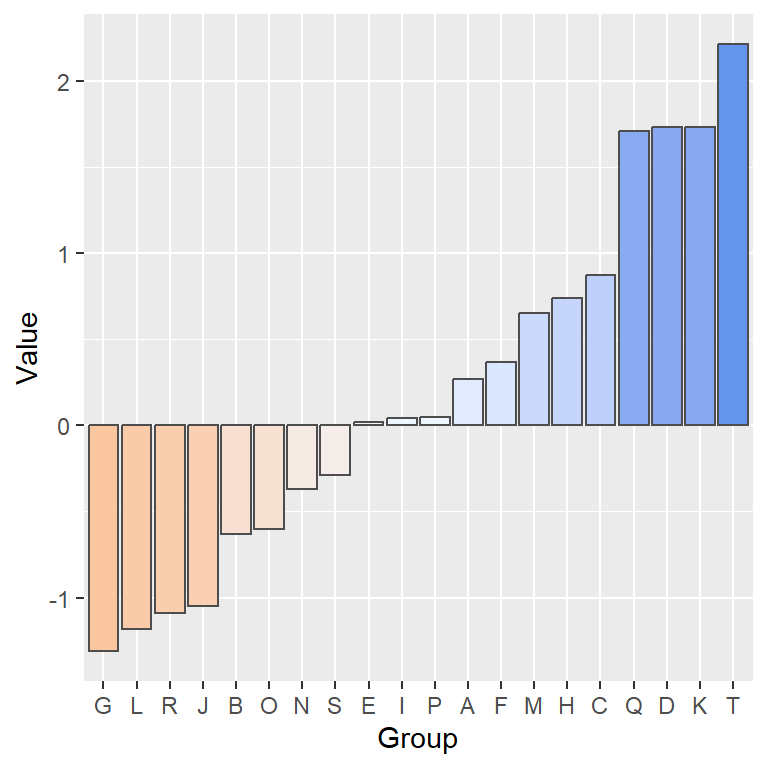## Advanced diverging bar plot in ggplot2

Finally, we are going to create a couple of more advanced examples of diverging bar charts in ggplot2, customizing the theme, the colors, the axes limits and the grid.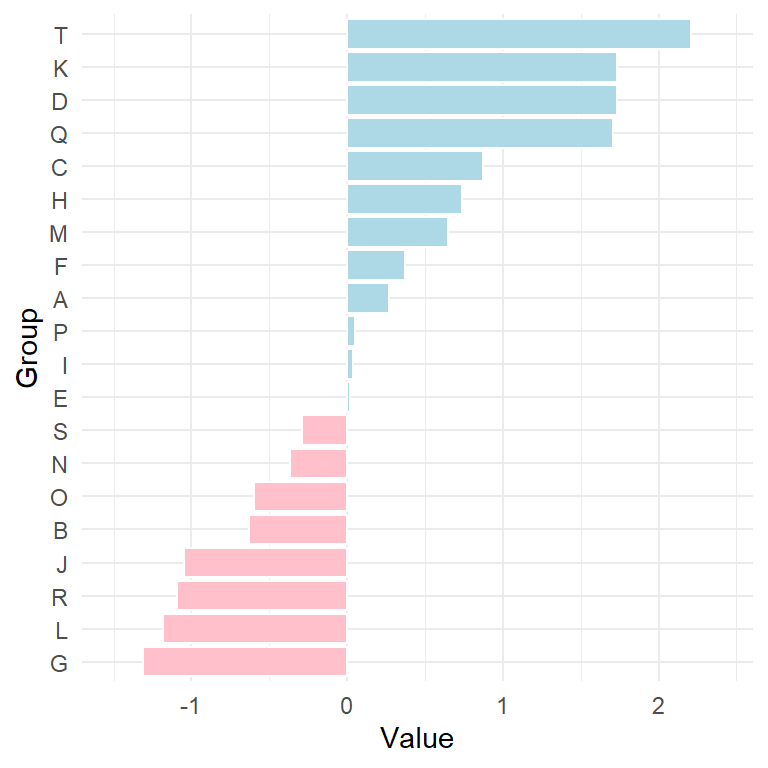Example 1: axis limits, color and theme

``````# install.packages("ggplot2")
library(ggplot2)

# Color based on value
color <- ifelse(df\$value < 0, "pink", "lightblue")

ggplot(df, aes(x = reorder(group, value), y = value)) +
geom_bar(stat = "identity",
show.legend = FALSE,
fill = color,      # Background color
color = "white") + # Border color
xlab("Group") +
ylab("Value") +
scale_y_continuous(breaks= seq(-2, 2, by = 1),
limits = c(min(df\$value) - 0.2,
max(df\$value) + 0.2)) +
coord_flip() +
theme_minimal() # Theme``````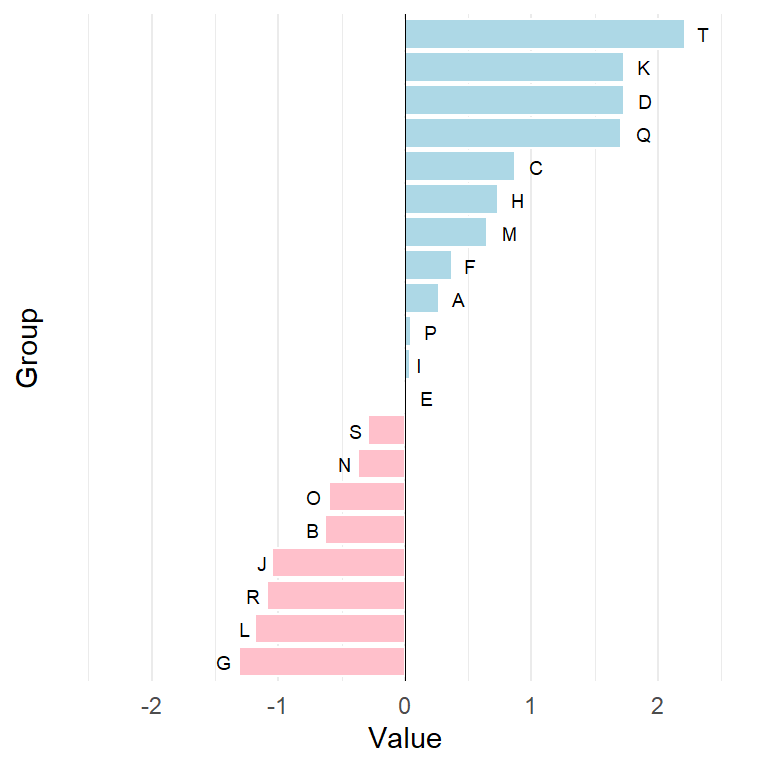Example 2: names for each bar, vertical grid and vertical line

``````# install.packages("ggplot2")
library(ggplot2)

# Color based on value
color <- ifelse(df\$value < 0, "pink", "lightblue")

ggplot(df, aes(x = reorder(group, value), y = value)) +
geom_bar(stat = "identity",
show.legend = FALSE,
fill = color,
color = "white") +
geom_hline(yintercept = 0, color = 1, lwd = 0.2) +
geom_text(aes(label = group, # Text with groups
hjust = ifelse(value < 0, 1.5, -1),
vjust = 0.5), size = 2.5) +
xlab("Group") +
ylab("Value") +
scale_y_continuous(breaks = seq(-2, 2, by = 1),
limits = c(-2.5, 2.5)) +
coord_flip() +
theme_minimal() +
theme(axis.text.y = element_blank(),  # Remove Y-axis texts
axis.ticks.y = element_blank(), # Remove Y-axis ticks
panel.grid.major.y = element_blank()) # Remove horizontal grid``````